# NLP学习第6讲

• 前馈神经网络、网络层数、输入层、隐藏层、输出层、隐藏单元、激活函数的概念。
• 感知机相关；定义简单的几层网络（激活函数sigmoid），递归使用链式法则来实现反向传播。
• 激活函数的种类以及各自的提出背景、优缺点。（和线性模型对比，线性模型的局限性，去线性化）
• 深度学习中的正则化（参数范数惩罚：L1正则化、L2正则化；数据集增强；噪声添加；early stop；Dropout层）、正则化的介绍。
• FastText的原理。
• 利用FastText模型进行文本分类。
• fasttext1 fasttext2 fasttext3 其中的参考

1.前馈网络包括一个输入层和一个输出层，若干隐单元。隐单元可以分层也可以不分层，若分层，则称为多层前馈网络。网络的输入、输出神经元其激活函数一般取为线性函数，而隐单元则为非线性函数。任意的前馈网络,不一定是分层网络或全连接的网络。

2. 网络层数是指神经网络中的层级。

3. 输入层是指神经网络中的输入参数这一层这是神经网络的第0层。

4. 隐藏层是指除输入层和输出层以外的其他各层叫做隐藏层。隐藏层不直接接受外界的信号,也不直接向外界发送信号。

5. 输出层是指最终结果的输入结果这一层，也是神经网络最后一层。

6. 隐藏单元是指在隐藏层中一个神经元。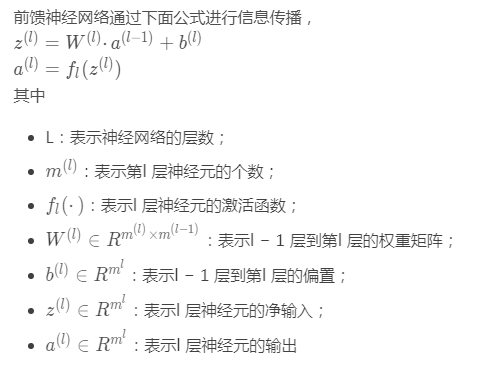1.基本原理在李航的《统计学习方法》里有，这里参考博客2的一段minist手写识别代码

# Import MNIST data，准备MNIST输入数据
from tensorflow.examples.tutorials.mnist import input_data

import tensorflow as tf

# Hyper Parameters，超参
learning_rate = 0.1
num_steps = 500
batch_size = 128
display_step = 100

# Network Parameters，网络参数
n_hidden_1 = 256 # 1st layer number of neurons
n_hidden_2 = 256 # 2nd layer number of neurons
num_input = 784 # MNIST data input (img shape: 28*28)
num_classes = 10 # MNIST total classes (0-9 digits)

# tf Graph input，TensorFlow图模型结构的输入定义
X = tf.placeholder("float", [None, num_input])
Y = tf.placeholder("float", [None, num_classes])

# Store layers weight & bias，定义需要学习的网络参数，即网络权值
weights = {
'h1': tf.Variable(tf.random_normal([num_input, n_hidden_1])),
'h2': tf.Variable(tf.random_normal([n_hidden_1, n_hidden_2])),
'out': tf.Variable(tf.random_normal([n_hidden_2, num_classes]))
}
biases = {
'b1': tf.Variable(tf.random_normal([n_hidden_1])),
'b2': tf.Variable(tf.random_normal([n_hidden_2])),
'out': tf.Variable(tf.random_normal([num_classes]))
}

# Create model，定义神经网络基本结构
def neural_net(x):
# Hidden fully connected layer with 256 neurons
# Hidden fully connected layer with 256 neurons
# Output fully connected layer with a neuron for each class
out_layer = tf.matmul(layer_2, weights['out']) + biases['out']
return out_layer

# Construct model，初始化所定义神经网络结构的实例
logits = neural_net(X)

# Define loss and optimizer，定义误差函数与优化方式
loss_op = tf.reduce_mean(tf.nn.softmax_cross_entropy_with_logits(
logits=logits, labels=Y))
train_op = optimizer.minimize(loss_op)

# Evaluate model (with test logits, for dropout to be disabled)，定义检验模型效果的方式
correct_pred = tf.equal(tf.argmax(logits, 1), tf.argmax(Y, 1))
accuracy = tf.reduce_mean(tf.cast(correct_pred, tf.float32))

# Initialize the variables (i.e. assign their default value)，初始化所有定义的网络变量
init = tf.global_variables_initializer()

# Start training，开启session，将所有定义编译成实际的TensorFlow图模型并运行
with tf.Session() as sess:

# Run the initializer
sess.run(init)

for step in range(1, num_steps+1):
batch_x, batch_y = mnist.train.next_batch(batch_size)
# Run optimization op (backprop)，进行前向传播，后向传播，以及优化
sess.run(train_op, feed_dict={X: batch_x, Y: batch_y})
if step % display_step == 0 or step == 1:
# Calculate batch loss and accuracy，计算每一批数据的误差及准确度
loss, acc = sess.run([loss_op, accuracy], feed_dict={X: batch_x,
Y: batch_y})
print("Step " + str(step) + ", Minibatch Loss= " +                   "{:.4f}".format(loss) + ", Training Accuracy= " +                   "{:.3f}".format(acc))

print("Optimization Finished!")

# Calculate accuracy for MNIST test images，计算测试数据上的准确度
print("Testing Accuracy:",         sess.run(accuracy, feed_dict={X: mnist.test.images,
Y: mnist.test.labels}))



import tensorflow as tf

input_data = tf.Variable( [[0, 10, -10],[1,2,3]] , dtype = tf.float32 )

output = tf.nn.sigmoid(input_data)

with tf.Session() as sess:

init = tf.initialize_all_variables()

sess.run(init)

print(sess.run(output))


static inline float linear_activate(float x){return x;}
static inline float logistic_activate(float x){return 1./(1. + exp(-x));}
static inline float relu_activate(float x){return x*(x>0);}
static inline float tanh_activate(float x){return (exp(2*x)-1)/(exp(2*x)+1);}

1.过拟合：经典图片，就是在已有的训练集训练的太好了，导致泛化性能很差。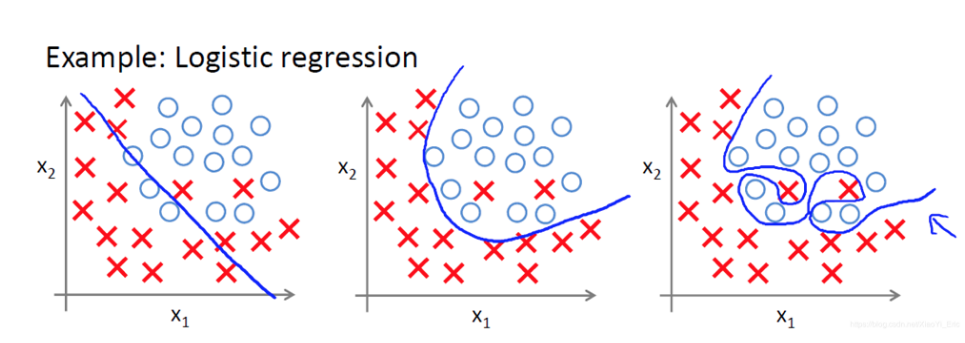2.正则化

（1）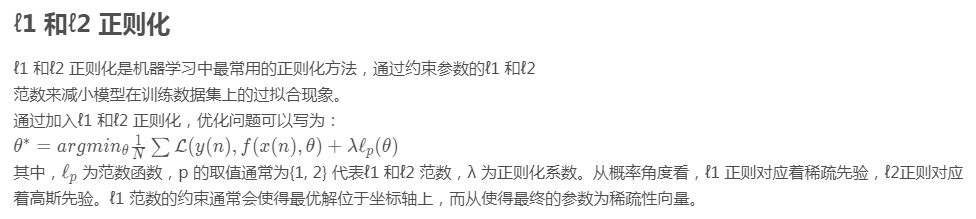（2）数据集增强

• 旋转（Rotation）：将图像按顺时针或逆时针方向随机旋转一定角度；
• 翻转（Flip）：将图像沿水平或垂直方法随机翻转一定角度；
• 缩放（Zoom In/Out）：将图像放大或缩小一定比例；
• 平移（Shift）：将图像沿水平或垂直方法平移一定步长；
• 加噪声（Noise）：加入随机噪声。

（3）early stop

（4）dropout

1.参数初始化

2.自适应学习率算法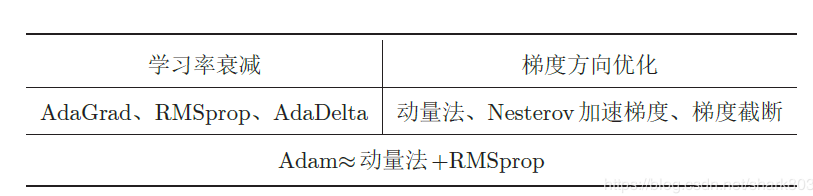2.batch norm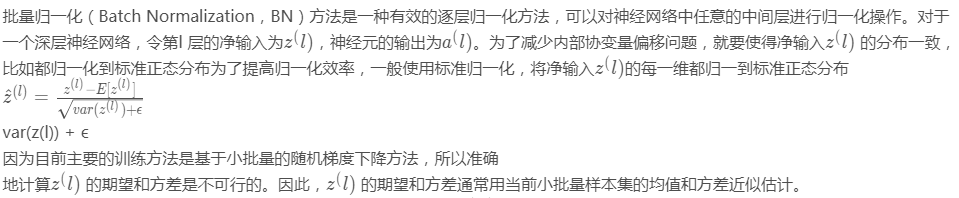3.layer norm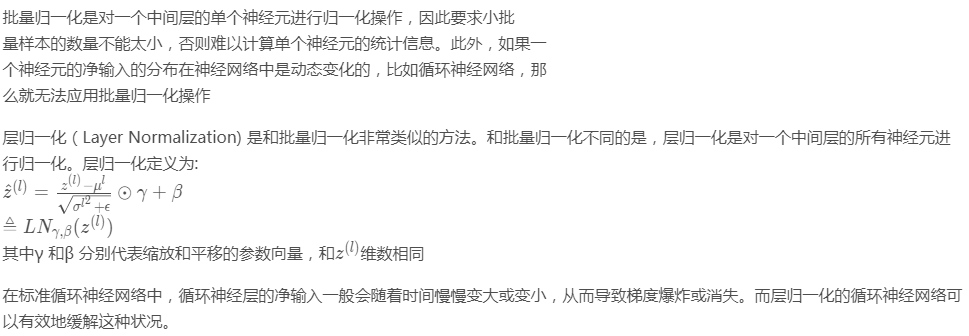https://blog.csdn.net/nc514819873/article/details/89431171

https://blog.csdn.net/shark803/article/details/89416013

https://blog.csdn.net/cs123456789dn/article/details/88408553

https://blog.csdn.net/mxs1123/article/details/88411283

©️2019 CSDN 皮肤主题: 猿与汪的秘密 设计师: 上身试试